Next: Cuts and Connectivity Up: Spanning Trees Previous: MAXIMUM MINIMUM SPANNING TREE   Index

• INSTANCE: Graph G=(V,E), three edge weight functions(for each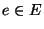), where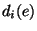denotes the weight of edge e if i of its endpoints are upgraded'', vertex upgrade costs c(v) (for each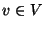), a threshold value D for the weight of a minimum spanning tree.

• SOLUTION: An upgrading set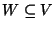of vertices such that the weight of a minimum spanning tree in G with respect to edge weights given byis bounded by D. Here,denotes the edge weight function resulting from the upgrade of the vertices in W, i.e.,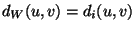where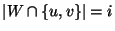.

• MEASURE: The cost of the upgrading set, i.e.,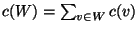.

• Good News: Approximable within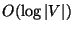if the difference of the largest edge weight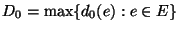and the smallest edge weightis bounded by a polynomial in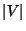.

• Bad News: At least as hard to approximate as MINIMUM DOMINATING SET .

• Comment: Variation in which the upgrading set must be chosen such that the upgraded graph contains a spanning tree in which no edge has weight greater than D is approximable within. In this case no additional assumptions about the edge weights are necessary. This variation of the problem is also at least as hard to approximate as MINIMUM DOMINATING SET .Next: Cuts and Connectivity Up: Spanning Trees Previous: MAXIMUM MINIMUM SPANNING TREE   Index
Viggo Kann
2000-03-20